SYLLABUS  Previous: 1.3.3 Dispersion  Up: 1.3 Prototype problems  Next: 1.3.5 Schrödinger

### 1.3.4 Wave-breaking

Slide : [ wave-breaking - shock-waves - solitons || VIDEO login]

Wave-breaking is a non-linearity that is particularly nicely understood when surfing on a see shore, where shallow waters steepen the waves until they break. The process can be modeled theoretically from the advection equation by choosing the velocity proportional to the amplitude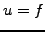: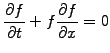(1.3.4#eq.1)

Since a local maximum (large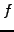) propagates faster than a local minimum (small), the top of the wave tries to over-take the bottom. The function soon becomes multi-valued causing the wave (and our numerical schemes) to break. Sometimes, the wave-breaking is balanced by a competing mechanism. This is the case of example in the Burger equation for shock-waves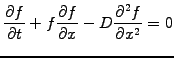(1.3.4#eq.2)

where the creation of a shock front (with short wavelengths) is physically limited by diffusion, which damps the short wavelengths (1.3.2#eq.4). Here is an example of a shock formation computed using a 2-levels explicit finite difference scheme from chapter 2.

 Numerical experiments: shock waves Vary the Diffusion parameter in the interval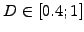where the results are more or less satisfactory at the beginning of the simulation when the time reaches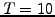. Click in the plot window to measure the propagation speed and count the density of mesh points to evaluate the steepness of the shock front as a function of the diffusion. Observe what happens if the Diffusion parameter is chosen outside the interval. Without spending much effort on a topic that will be discussed further soon, try to modify the simulation parameters to perform a physically relevant simulation using a small Diffusion=0.1.

Another type of non-linear equations where the wave-breaking is balanced by dispersion leads to the Korteweg-DeVries equation for solitons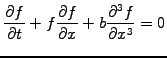(1.3.4#eq.3)

The evolution below shows how large amplitudes solitons (short wavelengths) propagate faster than lower amplitudes (long wavelength), in agreement with the dispersion analysis previously performed in sect.1.3.3.

 Numerical experiments: solitons Study the collision that occurs when the time reaches around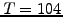. Monitor precisely the peak of each pulse as a function of time, to verify that solitons in fact never get superposed - even if the fast pulse ends up in front and eventually propagates independently of the slower pulse. Initialize a Gaussian pulse and observe how it decays into a so-called train of solitons that undergo multiple collisions in a periodic domain.

SYLLABUS  Previous: 1.3.3 Dispersion  Up: 1.3 Prototype problems  Next: 1.3.5 Schrödinger